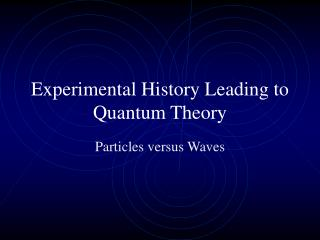DownloadDownload PresentationExperimental History Leading to Quantum Theory

# Experimental History Leading to Quantum Theory

Download Presentation## Experimental History Leading to Quantum Theory

- - - - - - - - - - - - - - - - - - - - - - - - - - - E N D - - - - - - - - - - - - - - - - - - - - - - - - - - -
##### Presentation Transcript

1. Experimental History Leading to Quantum Theory Particles versus Waves

2. Properties of Light~1650 • Travels in straight lines in a given material • Casts sharp shadows • Comes in colors • Reflects from smooth surfaces • Refracts when traveling from one material to another • Research limited to descriptions of “how” light behaved • In 1660’s, question changes to “why” light behaves as it does and to what is the fundamental nature of light

3. Model #1Isaac Newton & Particle Model • Light is a stream of small particles • Model adequately explains reflection & refraction • Colors produced by particles of different shapes • Behavior of particles governed by law of gravity • Predicts that light speeds up when going from air into a refracting medium

4. Model #2Christian Huygens & Wave Model • Light is a wave and each point on a wavefront serves as point sources of subsequent wavelets • Adequately explains reflection & refraction • Colors correspond to different wavelengths • Predicts that light slows down when going from air into refracting medium

5. Setting up the Problem • Is light a particle or a wave?

6. Resolving the DilemmaWhat is the speed of light? • Galileo ~ 1600 • Speed of light is very fast – too fast to measure • Roemer - 1676 • Used occultation of Io by Jupiter • Speed of light is finite, but very fast • Fizeau and Foucault – 1840’s • Measured speed of light in air and water • Found it to slow down in water

7. New Experimental ResultsYoung’s Double Slit Experiment • Interference fringes can only be explained with the wave model • Provided first technique for measuring wavelength of visible light

8. Merger of Optics and E&M • Maxwell unifies experiment work of Faraday, Ampere and Oersted into four laws expressed in vector calculus notation known today as Maxwell’s equations • Simultaneous solution results in an equation known to describe wave behavior, with the speed of the wave in vacuum being 3 x 108 m/s

9. Conclusion? • Newton was wrong • Light is a wave of electromagnetic origin • Wavelengths extend beyond the visible into ultraviolet and infrared regions • Waves carry energy • In 1880’s, physics is declared a dead science – the physical world is totally known • All that’s left are details and applications

10. One of the “Details” • What determines the colors emitted by an ideal radiator as it heats up? • Max Planck and blackbody radiation • A solid can be modeled as a matrix of small masses connected to nearest neighbors by springs • Vibrational modes of the springs dictate what wavelengths are emitted

11. Problems with Classical Explanation • Classically, vibrational modes form a continuum • Classical explanation leads to ultraviolet catastrophe • Planck’s resolution: only discrete, quantized vibrational modes are possible • E = nhf, where h is an empirical constant and n = 0, 1, 2…

12. Another “Detail”The Photoelectric Effect • When a polished metal surface is exposed to ultraviolet light, electrons (a.k.a. photoelectrons) are emitted from the surface with measurable kinetic energy

13. Experimental results Increased UV intensity results in larger number of emitted photoelectrons Increased UV frequency results in larger kinetic energy of photoelectrons Classical predictions Increased UV intensity results in larger kinetic energy of photoelectrons Increased UV frequency results in larger number of emitted photoelectrons Another Problem with Classical Physics

14. Einstein’s Explanation • Light comes in packets, called photons • Intensity is proportional to the number of photons • Energy of each photon is proportional to the frequency: E = hf • Result: 1921 Nobel Prize

15. Further Evidence • The Compton Effect • Photons of light carry momentum as well as energy • Result: 1927 Nobel Prize

16. The Real Problem • How can light be both a wave and a particle? • deBroglie’s response: If a wave can behave like a particle, then a particle can behave like a wave • Result: 1929 Nobel Prize

17. Experimental Confirmation • Davisson-Germer Experiment • When electrons pass through a crystalline lattice, they interfere to form an interference pattern similar to Young’s double slit experiment with light • Result: 1937 Nobel Prize

18. What’s Going On? • Are photons like particles or like waves? • Are particles like waves or like particles?